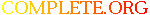Complete.Org: Mailing Lists: Archives: freeciv-data: August 2000: [freeciv-data] CivLog# [freeciv-data] CivLog

[Top] [All Lists]

 To: "Freeciv-Dev" , "Freeciv-Data" , "Freeciv" Subject: [freeciv-data] CivLog From: "Wong TM (Huang Deming)" Date: Thu, 3 Aug 2000 18:17:32 +0800 Reply-to: freeciv-data@xxxxxxxxxxx

```Welcome to CivLog 0.02Me
Me means 'Millenium Edition' :-)
Lots of new features and we decided to
leave the old bugs in there. The only thing
we took out of our Millenium Edition was the
DOZE - Mode. :-)

--extract from the JOODA team

Actually, it's a Java's Freeciv score log file reader and presents it using a
power
graph.

I developed this program so that I can analyse how I played versus the rest of
the
players/AI and see how and what's mine & others styles of playing Freeciv.

With this program, you can evaluate your performance and hopefully see where you
had fallen behind and learn from your mistake and correct it and make you a
better
player. You can also use it to see how well the AIs progress in the game.

You can find the full source code and binary package in
<ftp://ftp.freeciv.org/freeciv/incoming/CivLog-0.02ME.zip>.

attach is the source code only

btw

/** this formula calculate the no. of turn between any two freeciv years (>=
-4000)
*/
/** -4000 <= x < -1000, 50 turns */
turns =  Math.abs ( (Math.min (Math.max (startYear,-4000), -999)
- Math.min (Math.max (endYear,  -4000), -999)) / 50);
/** -1000 <= x < -1, 25 turns */
turns += Math.abs ( (Math.min (Math.max (startYear,-1000),   -1)
- Math.min (Math.max (endYear,  -1000),   -1)) / 25);
/** 0 <= x < 1000, 20 turns */
turns += Math.abs ( (Math.min (Math.max (startYear,    0),  999)
- Math.min (Math.max (endYear,      0),  999)) / 20);
/** 1000 <= x < 1500, 10 turns */
turns += Math.abs ( (Math.min (Math.max (startYear, 1000), 1499)
- Math.min (Math.max (endYear,   1000), 1499)) / 10);
/** 1500 <= x < 1750, 5 turns */
turns += Math.abs ( (Math.min (Math.max (startYear, 1500), 1749)
- Math.min (Math.max (endYear,   1500), 1749)) / 5);
/** 1750 <= x < 1900, 2 turns */
turns += Math.abs ( (Math.min (Math.max (startYear, 1750), 1899)
- Math.min (Math.max (endYear,   1750), 1899)) / 2);
/** 1900 <= x, 1 turn */
turns += Math.abs ( (Math.max (startYear, 1900) - Math.max (endYear, 1900)) /
1);
/** add a +1 to this formula to be more accurate */

Credits
=======
Raimar Falke <rf13@xxxxxxxxxxxxxxxxxxxxxxxx> For solving mine worst headache in
designing a
class holding the main CivLog data structure. You been a great help.
--
I Love Linux

-- Binary/unsupported file stripped by Listar --
-- Type: application/octet-stream
-- File: CivLog-0.02MEsrc.tar.bz2

```